Ground Penetrating Radar Frequency RangeX

# Ground Penetrating Radar Frequency Range

Frequency selection is controlled by two survey requirements – exploration depth and resolution length, as shown in Figure 1. Resolution length indicates the ability to uniquely identify closely spaced targets. More details on resolution length can be found in the January 2003 EKKO_Update.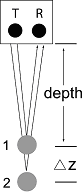Figure 1: Frequency selection is controlled by exploration depth and resolution length, D Z.

Exploration depth depends on many site specific factors with the most important being the signal attenuation rate in the host material. Attenuation rate depends on GPR frequency as indicated in Figure 2.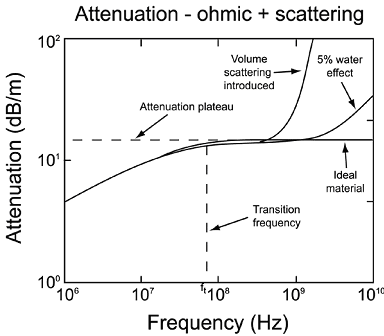Figure 2: Attenuation dictates exploration depth. In an ideal material, attenuation plateaus above the transition frequency. In real environments, water or volume scattering cause attenuation to increase with frequency. The on-set of high frequency losses is very site specific.

In an ideal material, attenuation plateaus at high frequency. In real materials, heterogeneity and water relaxation absorption increase attenuation at high frequency. Scattering losses, as illustrated in Figure 3, always occur. A street lamp in fog is a good analogy. Water drops scatter the light resulting in greatly reduced visibility (i.e. light penetration is decreased).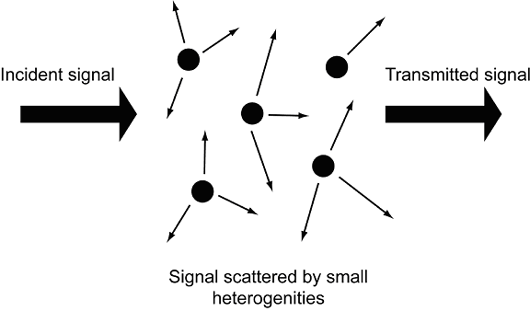Figure 3: GPR signals are scattered by small heterogeneities in material properties which reduce the transmitted signal.

Resolution length varies proportionately with GPR frequency since system bandwidth equals center frequency for impulse or baseband GPRs, as depicted in Figure 4.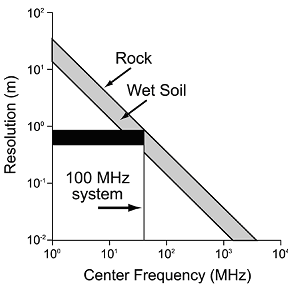Figure 4: Spatial resolution versus frequency length. Material velocity changes spatial resolution.

Figures 2 and 4 illustrate the dilemma: as GPR frequency increases, resolution increases but the exploration depth decreases. The compromise solution has a logical but not always unique solution.

Plotting exploration depth versus frequency, as shown in Figure 5, provides the basis of this discussion. For simplicity, exploration depth is selected to be three attenuation lengths in the material. Attenuation length is the inverse of attenuation rate and often referred to as skin depth.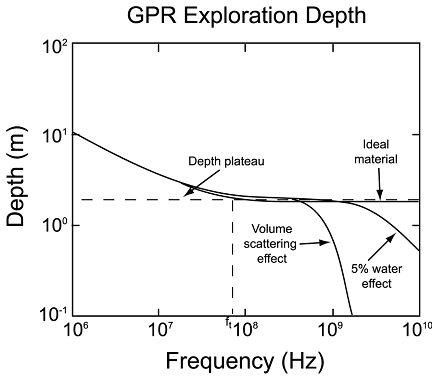Figure 5: Exploration depth (assumed to be three attenuation lengths) varies with frequency. The decrease in exploration depth at high frequency limits the upper practical GPR frequency.

As shown in Figure 6, the GPR bandwidth must lie between the shaded areas where GPR is not an appropriate method (dispersion is just too great). For maximum resolution fc is selected such that the upper edge of the GPR bandwidth touches the exploration depth curve. In some situations, a range of resolutions and center frequencies can be selected (Figure 7) while some situations leave little choice (Figure 8).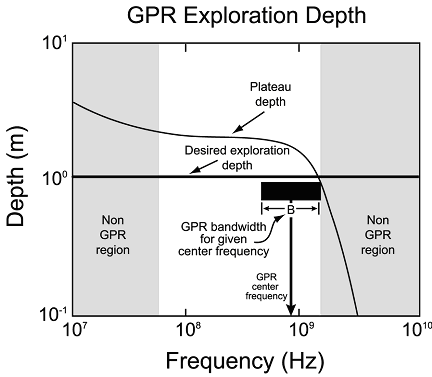Figure 6: On a log scale, GPR bandwidth and, hence, resolution increase and decrease as center frequency is changed. The highest resolution (smallest resolution length) is achieved when the upper edge of the bandwidth box touches the exploration depth curve at the desired exploration depth.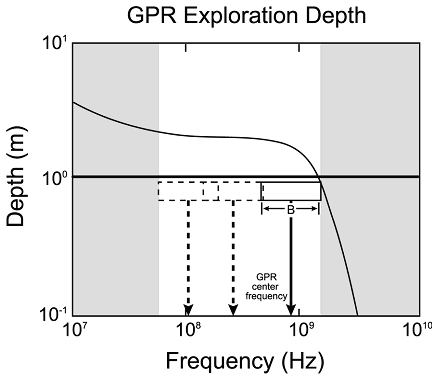Figure 7: The GPR frequency can be placed anywhere in the unshaded region, as depicted in the figure. As the center frequency is decreased, the bandwidth, B, decreases resulting in lower resolution.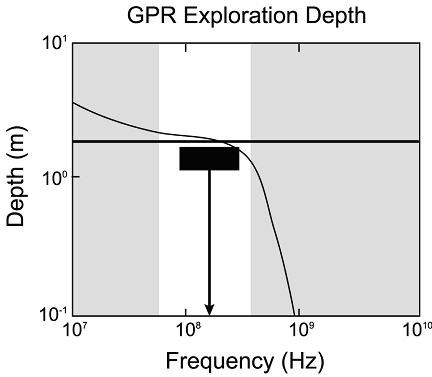Figure 8: In some cases, there is no choice about frequency, as shown here. As the heterogeneity scale length increases, the high frequency cut off moves lower.

The following is a simplified algorithm which can be coded in a spreadsheet and used to estimate fc based on this logic. (a) Characterize the site by estimating local relative permittivity, K, low frequency conductivity, , and heterogeneity scale, L (typical length of local variability in the host material). (b) Compute the exploration depth (see Figure 5).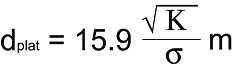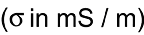(c) Specify the desired exploration depth, D (must be less than dplat). (d) Estimate high frequency limit factor for scattering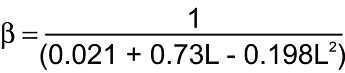(e) Estimate maximum resolution ratio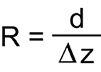using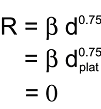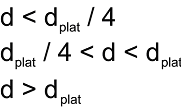(f) If R < 1, GPR is inappropriate. (g) If R > 1 then the GPR center frequency that gives the fairest depth versus resolution length compromise is: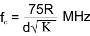If the host is very wet (high water content > 5%), then fc should be limited to less than 1500 MHz if the computed value is greater.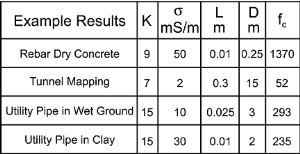These results are upper limits on frequency. Not included in this simple analysis is the fact that GPR system power and sensitivity tend to increase with decreasing frequency. Using a somewhat lower frequency than computed is often a wise choice.

﻿